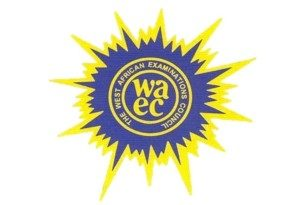###ONLINE CANDIDATES : 33### Confirmation Page# WAEC 2019 FURTHER MATHEMATICS OBJ & ESSAY VERIFIED ANSWERS(Further Mathematics Obj and Theory)

FURTHER MATHS OBJ:
1-10: AACACBDABC
11-20: DAACCDBDAA
21-30: BCCBABABCC
31-40: BCCBCCDDDD

Pay to get it early, does that paid got it earlier .

(2a)

Using y2 – y1/x2 – x1
Where y2 = 7, y1 = -5,
X2 = -2, and X1 = 7

7 – -5/-2 – 7
=7+5/-9
=12/-9
=4/-3
Coordinate points :
-4/3(3 : 2)
=-12/3 : -8/3
= -4 : -2⅔
X = (-4, 2⅔)

(2b)
2/1-√2 – 2/2+√2
=2(2+√2)-2(1-√2)/(1-√2)(2+√2)
=4+2√2 – 2+2√2/2+√2-2√2 – 2
=2 + 4√2/-√2
=(2+4√2)(-√2)/-√2(-√2)
= -2√2 – 4(2)/2
= -8 – 2√2/2
= -4 – √2

======================

No3

(3)
Sn = A/2[2n+(n-1)d]
Where Sn = 165
a = -3, d = 2
165 = A/2[2(-3)+(n-1)2]
165 = n[-6+2n-2] /2
165×2 = n[2n – 8]
330 = 2n² – 8n
2n² – 8n – 330 = 0
n²-4n-165 = 0

Using -b±√b²-4ac/2a
4±√-4²-4(1)(-165)/2(1)

4±√16 + 660/2
4±√676 = 4±26/2
4+26/2 = 30/2
= 15 terms
name

===============

No5

(5)
Draw the diagram

Using cosine law
Cos∅ = 16²+10²-14²/2(16)(10)

Cos∅ = 256 + 100 – 196/320

Cos∅ = 160/320
Cos∅ = 0.5
∅ = cos-¹(0.5)
∅ = 60°

Angle between 10N and 16N
= 180 – ∅ (sum of angles on a straight line)
= 180 – 60
=120°

==================

(6)
Draw the diagram
(T × 25)=(50×10)+(20×45)
25T = 500 + 900
25T = 1400
T = 1400/25
T = 56N

===============

No7

(7)
In a tabular form
Under class interval:
1-5, 6-10, 11-15, 16-20, 21-25, 26-30

Under class mark (X):
3, 8, 13, 18, 23, 28

Under X-Xbar:
-10, -5, 0, 5, 10, 15

Under frequency:
18, 12, 25, 15, 20, 10
Ef = 100

Under f(X – XbarA):
-180, -60, 0, 75, 200, 150
f(X – XbarA) = 185

Where xA = 13

Mean = xA + Ef(X – Xbar)/Ef
=13 + 185/100
=13 + 1.85
=14.85years

CHECK OUT:  WAEC SPECIMEN 2019/2020 PRACTICALS SOLUTIONS

===============

(4)
Draw the right angled triangle

Using Pythagoras theorem
Third side = √(p+q)² – (p-q)²
=√(p+q+p-q)(p+q-p+q)
Difference of two squares.
=√(2p)(2q)
=√4pq

Tanx = opp/adj = p – q/2√pq
1 – tan²X = 1-(p-q)²/4pq
=(4pq)-(p²-2pq+q²)/4pq
= -p²+6pq-q²/4pq
= -(p² – 6pq + q²)/4pq

=================

No9

(9a)
Y = 7 – 6/x and y + 2x – 3 = 0
Substitute eqn (1) into eqn(2)
7 – 6/x + 2x – 3 = 0
Multiply through by X
7x – 6 + 2x² – 3x = 0
2x² + 4x – 6 = 0
X² + 2x – 3 = 0
(x² + 3x – x – 3) = 0
X(X+3)-1(x+3)=0
(x-1)(x+3)=0
X – 1 = 0 or X + 3 = 0
X = 1 or X = -3
But y = 7 – 6/x
When X = 1
y = 7 – 6/1
y = 7 – 6
y = 1

When X = -3
y = 7 – 6/-3
y = 7 + 2
y = 9
Coordinates are (1, 1) and (-3, 9)

(9b)
Draw the diagram

Gradient of AB = 9 – 1/-3 -1
=8/-4 = -2
Midpoint of AB = (1+3/2, 1-9/2) = (2, -4)
Gradient of perpendicular = -1/-2 = 1/2

equation of perpendicular is
y-(-4)/x-2 = 1/2
y + 4/x – 2 = 1/2
y = 1/2x – 1 – 4
y = 1/2x – 5
OR
2y = X – 10

==============

(10a)
Given 4x² – px +1 = 0
For real roots: b² – 4ac >0
(-p) ² – 4(4) (1) > 0
p² – 16 > 0
p² >16
p > ± 4

(10bi)
Given: (1 +3x)⁶
Using pascal’s triangle: 1, 6, 15, 20, 15, 6, 1
(1)⁶(3x)º + 6(1)⁵ (3x)¹ + 15 (1)⁴ (3x)₂ + 20(1)³ (3x)³ + (15) (1)²(3x)⁴+6(1)¹(3x)⁵ + 1(1)º(3x)⁶
1 + 6(3x) + 15 (9x²) + 20 (27x³) + 15 (81x⁴) + 6(243 x⁵) 729x⁶
1 + 18x + 135x² + 540x³ + 1215x⁴ 1458x⁵ + 729x⁶

(10bii)
(1.03)⁶ = (1 + 3(0.01)]
Therefore (1.03)⁶ = 1 + 18(0.01) + 135 (0.01)² + 540(0.01)³ + 1215(0.01)⁴
+ 1458 (0.01)⁵ + 729 (0.01)⁶
+ 1 + 0.18 + 0.0135 + 0.005 + 0.00001215
+ 0.0000001458 + 0.000000000729
= 1.1940523
= 1.194 (4s.f)

==================

11)

u= 5ms-1                                   u= 6ms-1
A= 1ms-2                                     a= 3ms-2
p→                                                        ←a

M—————————————N
51m

11a)t when particles are 30 meters apart
S= ut + ½ at2
Sp= st + ½ t2
Sa = 6t + 1/2 (3)t2
Where sp and sa are distances covered by particles respectively at any time t
At time, t= 1.5s
Sp = 5(1.5) +  ½(1.5)2 =8.625m
sa = 6(1.5) + ½ (3)(1.5)2 = 12.375m
sp + sa = 8.625 + 12.375
= 21m
Distance between particles = 51 – 21 = 30m
At time t = 1.5s, the particles will be 30 meters apart.

CHECK OUT:  LIST OF OUR EXAM RUNS PACKAGES AND THEIR EXPLANATIONSJoin Our Telegram Channel |Whatsapp Our Admins: Click Any Name Mr.Paul or Mr.Henry

### Comment

Posted by on 3rd May 2019.

Categories: WAEC